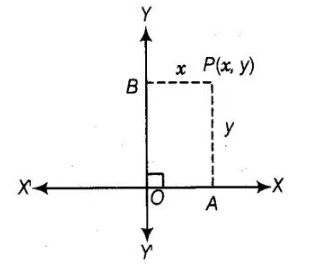# The distance of the point P(2, 3)

Question:

The distance of the point P(2, 3) from the X-axis is

(a) 2

(b) 3

(c) 1

(d) 5

Solution:

(b) We know that, if (x, y) is any point on the cartesian plane in first quadrant.

Then, x = Perpendicular distance from Y-axis

and y = Perpendicular distance from X-axisDistance of the point P(2, 3) from the X-axis = Ordinate of a point P(2, 3)= 3.